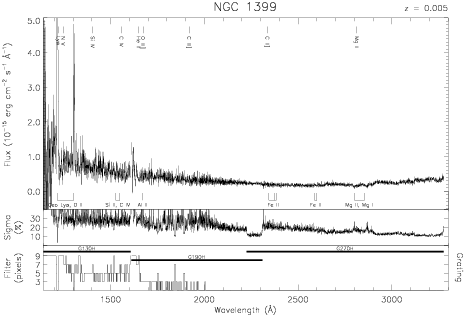Download dataset
TELESCOP= 'HST     '
INSTRUME= 'FOS     '
RADECSYS= 'FK5     '
EQUINOX =               2000.0
DATE-OBS= '1993-01-17T04:02:32'
RA_PNT  =        54.6208333333
DEC_PNT =       -35.4500000000
PA_PNT  =       196.3870613187
OBJECT  = 'NGC 1399'
ROOTNM01= 'Y15I0102T'
GRNDMD01= 'SPECTROSCOPY'
DETECT01= 'AMBER   '
APERID01= 'B-3     '
FGWAID01= 'H19     '
ROOTNM02= 'Y15I0103T'
GRNDMD02= 'SPECTROSCOPY'
DETECT02= 'AMBER   '
APERID02= 'B-3     '
FGWAID02= 'H19     '
ROOTNM03= 'Y15I0104T'
GRNDMD03= 'SPECTROSCOPY'
DETECT03= 'AMBER   '
APERID03= 'B-3     '
FGWAID03= 'H19     '
ROOTNM04= 'Y15I0105T'
GRNDMD04= 'SPECTROSCOPY'
DETECT04= 'AMBER   '
APERID04= 'B-3     '
FGWAID04= 'H27     '
ROOTNM05= 'Y15I0106T'
GRNDMD05= 'SPECTROSCOPY'
DETECT05= 'AMBER   '
APERID05= 'B-3     '
FGWAID05= 'H27     '
ROOTNM06= 'Y15I0202T'
GRNDMD06= 'SPECTROSCOPY'
DETECT06= 'BLUE    '
APERID06= 'B-3     '
FGWAID06= 'H13     '
ROOTNM07= 'Y15I0203T'
GRNDMD07= 'SPECTROSCOPY'
DETECT07= 'BLUE    '
APERID07= 'B-3     '
FGWAID07= 'H13     '
ROOTNM08= 'Y15I0204T'
GRNDMD08= 'SPECTROSCOPY'
DETECT08= 'BLUE    '
APERID08= 'B-3     '
FGWAID08= 'H13     '
ROOTNM09= 'Y15I0205T'
GRNDMD09= 'SPECTROSCOPY'
DETECT09= 'BLUE    '
APERID09= 'B-3     '
FGWAID09= 'H13     '
ROOTNM10= 'Y15I0206T'
GRNDMD10= 'SPECTROSCOPY'
DETECT10= 'BLUE    '
APERID10= 'B-3     '
FGWAID10= 'H13     '